#### Thank you for registering.

One of our academic counsellors will contact you within 1 working day.

Please check your email for login details.

Click to Chat

1800-1023-196

+91 7353221155

CART 0

• 0
MY CART (5)

Use Coupon: CART20 and get 20% off on all online Study Material

ITEM
DETAILS
MRP
DISCOUNT
FINAL PRICE
Total Price: Rs.

There are no items in this cart.
Continue Shopping

# How to do this ?Shouldn’t the answer be 0 since the net enclosed charge is 0 ?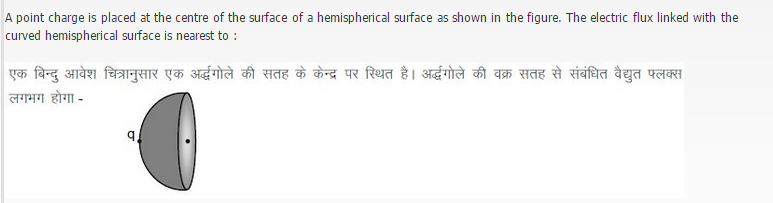## 1 Answers

6 years ago
Answer is not zero.  As the surface is not closed surface.  To solve this problem look at the picture below: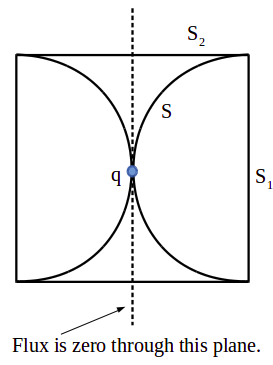p { margin-bottom: 0.25cm; line-height: 120%; }

Let us construct a cylinder as shown in the figure, with R (radius of hemisphere) is the radius of the cylinder and 2R is its length. Now you can see that the charge q will be at the center of the cylinder.

Let

S1 = end surface of the cylinder, φ1 = flux through S1

S2 = half of the curved surface of the cylinder, φ2 = flux through S2

S = is the curved surface of the hemisphere and φ = flux through S

Since flux through the central plane is zero, by symmetry we have φ! + φ2 = q/2εo

We can also see that φ = φ2 = (q/2εo)φ1

Since the surface subtends a plane angle of 90o at the q, the corresponding solid angle can be calculated using the formula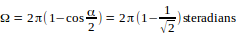, where α is the plane angle subtended.

Thus φ1 can be calculated as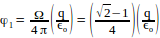Finally the required value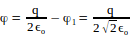## ASK QUESTION

Get your questions answered by the expert for free